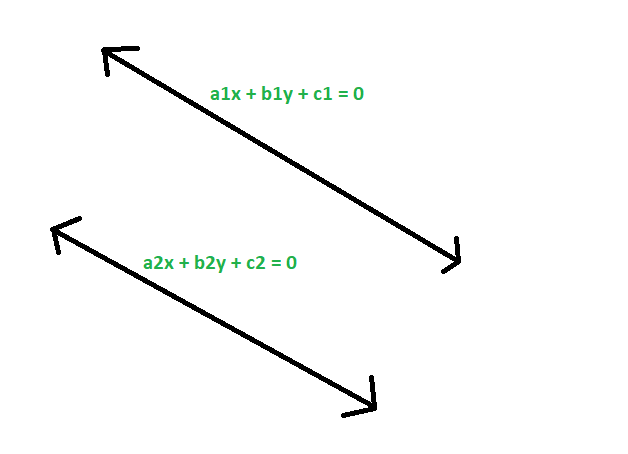GeeksforGeeks App
Open AppBrowser
Continue

# Check if given two straight lines are identical or not

Given two straight lines with co-efficients of their equations a1x + b1y + c1 = 0 and a2x + b2y + c2 = 0 respectively, the task is to check if the straight lines are identical or not.
Examples:

Input: a1 = -2, b1 = 4, c1 = 3, a2 = -6, b2 = 12, c2 = 9
Output: The given straight lines are identical
Input: a1 = 12, b1 = 3, c1 = 8, a2 = 7, b2 = -12, c2 = 0
Output: The given straight lines are not identicalApproach

1. Given equations,
a1x + b1y + c1 = 0
a2x + b2y + c2 = 0

2. converting them to slope intercept form, we get
y = (-a1/b1)x +(-c1/b1)
y = (-a2/b2)x +(-c2/b2)

3. now, if the lines are identical then there slope and intercepts must be equal,
so,
-a1/b1 = -a2/b2
or, a1/a2 = b1/b2
also,
-c1/b1 = -c2/b2
so, c1/c2 = b1/b2

4. So, if two given straight lines are identical then there co-efficients should be proportional.Below is the implementation of the above approach

## C++

 // C++ program to check if// given two straight lines// are identical or not #include using namespace std; // Function to check if they are identicalvoid idstrt(double a1, double b1,            double c1, double a2,            double b2, double c2){    if ((a1 / a2 == b1 / b2)        && (a1 / a2 == c1 / c2)        && (b1 / b2 == c1 / c2))        cout << "The given straight"             << " lines are identical"             << endl;     else        cout << "The given straight"             << " lines are not identical"             << endl;} // Driver Codeint main(){    double a1 = -2, b1 = 4,           c1 = 3, a2 = -6,           b2 = 12, c2 = 9;    idstrt(a1, b1, c1, a2, b2, c2);    return 0;}

## Java

 // Java program to check if// given two straight lines// are identical or notclass GFG{ // Function to check if they are identicalstatic void idstrt(double a1, double b1,                    double c1, double a2,                    double b2, double c2){    if ((a1 / a2 == b1 / b2)        && (a1 / a2 == c1 / c2)        && (b1 / b2 == c1 / c2))        System.out.println( "The given straight"        +" lines are identical");     else        System.out.println("The given straight"            + " lines are not identical");} // Driver Codepublic static void main(String[] args){    double a1 = -2, b1 = 4,            c1 = 3, a2 = -6,            b2 = 12, c2 = 9;    idstrt(a1, b1, c1, a2, b2, c2);}} // This code has been contributed by 29AjayKumar

## Python3

 # Python3 program to check if# given two straight lines# are identical or not # Function to check if they are identicaldef idstrt(a1, b1, c1, a2, b2, c2):    if ((a1 // a2 == b1 // b2) and        (a1 // a2 == c1 // c2) and        (b1 // b2 == c1 // c2)):        print("The given straight lines",                         "are identical");    else:        print("The given straight lines",                     "are not identical"); # Driver Codea1, b1 = -2, 4c1, a2 = 3,-6b2, c2 = 12,9idstrt(a1, b1, c1, a2, b2, c2) # This code is contributed# by mohit kumar

## C#

 // C# program to check if// given two straight lines// are identical or notusing System; class GFG{ // Function to check if they are identicalstatic void idstrt(double a1, double b1,                    double c1, double a2,                    double b2, double c2){    if ((a1 / a2 == b1 / b2)        && (a1 / a2 == c1 / c2)        && (b1 / b2 == c1 / c2))        Console.WriteLine( "The given straight"        +" lines are identical");     else        Console.WriteLine("The given straight"            + " lines are not identical");} // Driver Codepublic static void Main(String[] args){    double a1 = -2, b1 = 4,            c1 = 3, a2 = -6,            b2 = 12, c2 = 9;    idstrt(a1, b1, c1, a2, b2, c2);}} // This code contributed by Rajput-Ji

## PHP

 

## Javascript

 

Output:

The given straight lines are identical

Time Complexity: O(1)

Auxiliary Space: O(1)

My Personal Notes arrow_drop_up